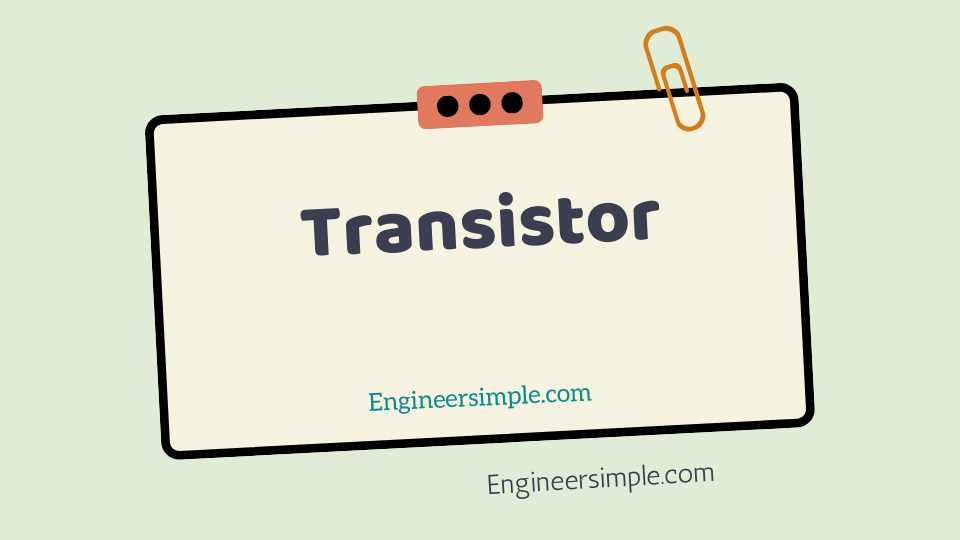Transistor - Engineer Simple

#A transistor consists of two pn junctions formed by *sandwiching either p-type or n-type
semiconductor between a pair of opposite types.

### Accordingly; there are two typws oftransistors, namely:

(i) n-p-n transistor (ii) p-n-p transistor

An n-p-n is composed of two n-type semiconductors separated by a
thin section of p-type as shown in Fig.1. However, a p-n-p is
formed by two p-sections separated by a thin section of n-type as shown in Fig.
1

### In each type of transistor, the following points may be noted:

(i) These are two pn junctions. Therefore, it may be regarded as a
combination of two diodes connected back to back.
(ii) There are three terminals, one taken from each type of seminconductor.
(iii) The middle section is a very thin layer. This is the most important factor in
the function of a transistor.
Origin of the name “Transistor”. When new devices are invent, scientists often
try to devise a name that will appropriately describe the device. A transistor has
two pn junctions.

As discussed later, one junction is forward bias and the
other is reverse bias. The forward biased junction has a low resistance path
whereas a reverse biased junction has a high resistance path. The weak signal is
introduced in the low resistance circuit and output is take from the high
resistance circuit. Therefore, it transfers a signal from a low resistance
to high resistance. The prefix ‘trans’ means the signal transfer property of the
device while ‘istor’ classifies it as a solid element in the same general famil with
resistors.
…………………………………………………………………………………………………………………………..